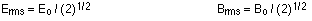## Electromagnetic waves

7-26-99

At this point in the course we'll move into optics. This might seem like a separate topic from electricity and magnetism, but optics is really a sub-topic of electricity and magnetism. This is because optics deals with the behavior of light, and light is one example of an electromagnetic wave.

### Light and other electromagnetic waves

Light is not the only example of an electromagnetic wave. Other electromagnetic waves include the microwaves you use to heat up leftovers for dinner, and the radio waves that are broadcast from radio stations. An electromagnetic wave can be created by accelerating charges; moving charges back and forth will produce oscillating electric and magnetic fields, and these travel at the speed of light. It would really be more accurate to call the speed "the speed of an electromagnetic wave", because light is just one example of an electromagnetic wave.

speed of light in vacuum: c = 3.00 x 108 m/s

As we'll go into later in the course when we get to relativity, c is the ultimate speed limit in the universe. Nothing can travel faster than light in a vacuum.

There is a wonderful connection between c, the speed of light in a vacuum, and the constants that appeared in the electricity and magnetism equations, the permittivity of free space and the permeability of free space. James Clerk Maxwell, who showed that all of electricity and magnetism could be boiled down to four basic equations, also worked out that: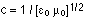This clearly shows the link between optics, electricity, and magnetism.

### Creating an electromagnetic wave

We've already learned how moving charges (currents) produce magnetic fields. A constant current produces a constant magnetic field, while a changing current produces a changing field. We can go the other way, and use a magnetic field to produce a current, as long as the magnetic field is changing. This is what induced emf is all about. A steadily-changing magnetic field can induce a constant voltage, while an oscillating magnetic field can induce an oscillating voltage.

Focus on these two facts:

1. an oscillating electric field generates an oscillating magnetic field
2. an oscillating magnetic field generates an oscillating electric field

Those two points are key to understanding electromagnetic waves.

An electromagnetic wave (such as a radio wave) propagates outwards from the source (an antenna, perhaps) at the speed of light. What this means in practice is that the source has created oscillating electric and magnetic fields, perpendicular to each other, that travel away from the source. The E and B fields, along with being perpendicular to each other, are perpendicular to the direction the wave travels, meaning that an electromagnetic wave is a transverse wave. The energy of the wave is stored in the electric and magnetic fields.

### Properties of electromagnetic waves

Something interesting about light, and electromagnetic waves in general, is that no medium is required for the wave to travel through. Other waves, such as sound waves, can not travel through a vacuum. An electromagnetic wave is perfectly happy to do that.

An electromagnetic wave, although it carries no mass, does carry energy. It also has momentum, and can exert pressure (known as radiation pressure). The reason tails of comets point away from the Sun is the radiation pressure exerted on the tail by the light (and other forms of radiation) from the Sun.

The energy carried by an electromagnetic wave is proportional to the frequency of the wave. The wavelength and frequency of the wave are connected via the speed of light: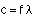Electromagnetic waves are split into different categories based on their frequency (or, equivalently, on their wavelength). In other words, we split up the electromagnetic spectrum based on frequency. Visible light, for example, ranges from violet to red. Violet light has a wavelength of 400 nm, and a frequency of 7.5 x 1014 Hz. Red light has a wavelength of 700 nm, and a frequency of 4.3 x 1014 Hz. Any electromagnetic wave with a frequency (or wavelength) between those extremes can be seen by humans.

Visible light makes up a very small part of the full electromagnetic spectrum. Electromagnetic waves that are of higher energy than visible light (higher frequency, shorter wavelength) include ultraviolet light, X-rays, and gamma rays. Lower energy waves (lower frequency, longer wavelength) include infrared light, microwaves, and radio and television waves.

### Energy in an electromagnetic wave

The energy in an electromagnetic wave is tied up in the electric and magnetic fields. In general, the energy per unit volume in an electric field is given by:In a magnetic field, the energy per unit volume is: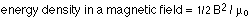An electromagnetic wave has both electric and magnetic fields, so the total energy density associated with an electromagnetic wave is: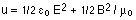It turns out that for an electromagnetic wave, the energy associated with the electric field is equal to the energy associated with the magnetic field, so the energy density can be written in terms of just one or the other:This also implies that in an electromagnetic wave, E = cB.

A more common way to handle the energy is to look at how much energy is carried by the wave from one place to another. A good measure of this is the intensity of the wave, which is the power that passes perpendicularly through an area divided by the area. The intensity, S, and the energy density are related by a factor of c: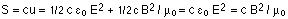Generally, it's most useful to use the average power, or average intensity, of the wave. To find the average values, you have to use some average for the electric field E and the magnetic field B. The root mean square averages are used; the relationship between the peak and rms values is: